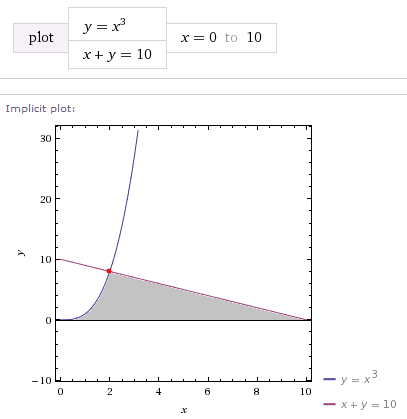# Find centroid two-dimensional region

## Homework Statement

Find the centroid of the region bounded by the given curves.

$$y = x^3$$$$x + y = 10$$$$y = 0$$

## Homework Equations

$$\bar x = \frac{1}{A}\int^b_a xf(x) dx$$$$\bar y = \frac{1}{A}\int^b_a \frac{1}{2}(f(x))^2 dx$$
Where A is the area of the region containing the centroid.

## The Attempt at a Solution

First thing I did was find the intersection of the curves $$x^3 = 10-x \Rightarrow x = 2 \Rightarrow P(2,8)$$
Then I put things in terms of y to integrate to find the area A of the region.$$y = x^3 \Rightarrow x = \sqrt y$$$$x + y = 10 \Rightarrow x = 10 - y$$$$A = \int^8_0 ((10-y)-(\sqrt y)) dy = 36$$
So $$\bar x = \frac{1}{A}\int^b_a yf(y) dy = \frac{1}{36}\int^8_0 y((10-y)-(\sqrt y))dy = \frac{496}{189} \approx 2.63$$$$\bar y = \frac{1}{A}\int^b_a \frac{1}{2}(f(y))^2 dy = \frac{1}{36}\int^8_0 \frac{1}{2}((10-y)^2-(\sqrt y)^2)dy = \frac{584}{135} \approx 4.33$$
So the centroid is at $$\left(\frac{496}{189},\frac{584}{135}\right)$$
What I'm getting here isn't correct. Thanks for commenting!

HallsofIvy
Science Advisor
Homework Helper

## Homework Statement

Find the centroid of the region bounded by the given curves.

$$y = x^3$$$$x + y = 10$$$$y = 0$$

## Homework Equations

$$\bar x = \frac{1}{A}\int^b_a xf(x) dx$$$$\bar y = \frac{1}{A}\int^b_a \frac{1}{2}(f(x))^2 dx$$
Where A is the area of the region containing the centroid.

## The Attempt at a Solution

First thing I did was find the intersection of the curves $$x^3 = 10-x \Rightarrow x = 2 \Rightarrow P(2,8)$$
Then I put things in terms of y to integrate to find the area A of the region.$$y = x^3 \Rightarrow x = \sqrt y$$$$x + y = 10 \Rightarrow x = 10 - y$$$$A = \int^8_0 ((10-y)-(\sqrt y)) dy = 36$$
This is completely wrong. If you look at a graph of x+y= 10 and $y= x^3$, then you will see that, for each y, x ranges from 0 on the left to
1)$y^{1/3}$ for y between 0 and 8 and
2) $10 - y$ for y between 8 and 10.
The area is given by
$$\int_{y= 0}^8 y^{1/3}dy+ \int_{y= 8}^{10} (10- y) dy$$.

Much simpler, and what most people would do, is to integrate with respect to x. For each x, between 0 and 2, y ranges from $x^3$ to $10- x$ so the area would be
$$\int_{x=0}^2(10- x- x^3) dx$$

So $$\bar x = \frac{1}{A}\int^b_a yf(y) dy = \frac{1}{36}\int^8_0 y((10-y)-(\sqrt y))dy = \frac{496}{189} \approx 2.63$$$$\bar y = \frac{1}{A}\int^b_a \frac{1}{2}(f(y))^2 dy = \frac{1}{36}\int^8_0 \frac{1}{2}((10-y)^2-(\sqrt y)^2)dy = \frac{584}{135} \approx 4.33$$
So the centroid is at $$\left(\frac{496}{189},\frac{584}{135}\right)$$
What I'm getting here isn't correct. Thanks for commenting!

Thank you for responding, HallsofIvy!

I think I'm still confused. I thought this was the region I needed to find the centroid of:Which has an area $$A = \int^2_0 x^3 dx + \int^{10}_2 (10-x) dx = 36$$

HallsofIvy
Science Advisor
Homework Helper
Oh, blast! I misead "y= 0" as "x= 0".

I had another look and figured it out. I guess when I did this in terms of y my x and y coordinates got flipped. The values of the integral are correct, but the coordinates are backwards, so the centroid is at$$\left(\frac{584}{135},\frac{496}{189}\right)$$